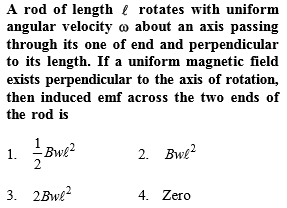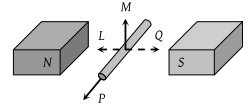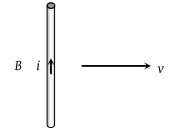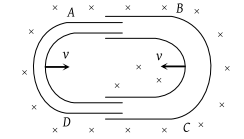You’ve reached the end of your free Videos limit.
#7 | Problem Set1: Motional EM
(Physics) > Electromagnetic Induction
Related Practice Questions :Complete Question Bank + Test Series
Complete Question Bank

Difficulty Level:

An electric potential difference will be induced between the ends of the conductor shown in the diagram, when the conductor moves in the direction(1) P

(2) Q

(3) L

(4) M

Complete Question Bank + Test Series
Complete Question Bank

Difficulty Level:

Two rails of a railway track insulated from each other and the ground are connected to a milli voltmeter. What is the reading of voltmeter, when a train travels with a speed of 180 km/hr along the track. Given that the vertical component of earth's magnetic field is 0.2 × 10–4 weber/m2 and the rails are separated by 1 metre

(1) 10–2 volt

(2) 10–4 volt

(3) 10–3 volt

(4) 1 volt

Complete Question Bank + Test Series
Complete Question Bank

Difficulty Level:

A conducting wire is moving towards right in a magnetic field B. The direction of induced current in the wire is shown in the figure. The direction of magnetic field will be(1) In the plane of paper pointing towards right

(2) In the plane of paper pointing towards left

(3) Perpendicular to the plane of paper and down wards

(4) Perpendicular to the plane of paper and upwards

Complete Question Bank + Test Series
Complete Question Bank

Difficulty Level:

One conducting U tube can slide inside another as shown in figure, maintaining electrical contacts between the tubes. The magnetic field B is perpendicular to the plane of the figure. If each tube moves towards the other at a constant speed v then the emf induced in the circuit in terms of B, l and v where l is the width of each tube, will be(1) Zero

(2) 2 Blv

(3) Blv

(4) – Blv